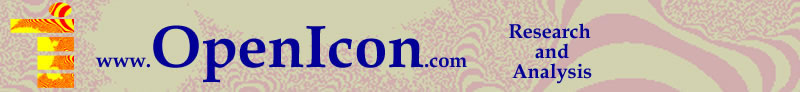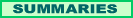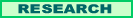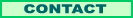### Visual Basic .NET Mandelbrot Set Fractal Program

####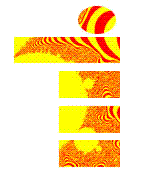The explicit instructions here assume you are using Microsoft Visual Basic .NET, which runs in Visual Studio, but if you code in another language it should not be too hard to do the same thing. You can use this program to produce bitmap graphics from a Mandelbrot Set, whether or not you are doing the rest of the project.

I did not try to construct a general purpose Mandelbrot program, or something with controls that allowed zooming in and out, moving on the X and Y axis, or changing the colors. I did all that by changing the code by hand. First I'll show the code in its entirety, then I'll make some comments on specific sections.

If you are a novice keep in mind that much of the code is generated by Microsoft Visual Studio when you create the form and button.

Public Class MandelbrotForm1

Private Sub MandelbrotForm1_Load(ByVal sender As System.Object, ByVal e As System.EventArgs) Handles MyBase.Load

End Sub

Private Sub GoButton_Click(ByVal sender As System.Object, ByVal e As System.EventArgs) Handles GoButton.Click
Dim myPen As New System.Drawing.Pen(System.Drawing.Color.White)
Dim MandelGraphics As System.Drawing.Graphics
Dim mBitmapGraphics As System.Drawing.Graphics
Dim myColor12, myColor13 As Color
Dim i, j
Dim x, y, x2, y2, x0, y0 As Double
Dim iter, maxit As Integer
Dim m_Bitmap As Bitmap
m_Bitmap = New Bitmap(500, 500)
MandelGraphics = PictureBox1.CreateGraphics
mBitmapGraphics = Graphics.FromImage(m_Bitmap)
myColor13 = Color.Yellow
myColor12 = Color.Red

myPen.Color = myColor1
maxit = 500
For i = 0 To 500
For j = 0 To 500
iter = 0
x = 0.225 + (i / 3000)
y = 0.43 + (j / 3000)
x0 = x
y0 = y
x2 = x * x
y2 = y * y
While (x2 + y2 < 8) And iter < maxit
y = (2 * x * y) + y0
x = x2 - y2 + x0
x2 = x * x
y2 = y * y
iter = iter + 1
End While
TextBox1.Text = iter
Select Case (iter Mod 2)
Case 1
myPen.Color = myColor12
Case 0
myPen.Color = myColor13
End Select
MandelGraphics.DrawLine(myPen, i, j, i + 1, j)
mBitmapGraphics.DrawLine(myPen, i, j, i + 1, j)
Next
Next
m_Bitmap.Save("C:\Documents and Settings\Bill\My Documents\Mandelbrot.bmp")
myPen.Dispose()
MandelGraphics.Dispose()
m_Bitmap.Dispose()
mBitmapGraphics.Dispose()
End Sub

Private Sub CaptureButton_Click(ByVal sender As System.Object, ByVal e As System.EventArgs)
Dim mGraphics As System.Drawing.Graphics
Dim m_Bitmap As Bitmap
m_Bitmap = New Bitmap(500, 500)
mGraphics = Graphics.FromImage(m_Bitmap)
m_Bitmap.Save("C:\Documents and Settings\Bill\My Documents\Mandelbrot")
m_Bitmap.Dispose()
mGraphics.Dispose()
End Sub
End Class

The centerpiece of this or any Mandlebrot graphic program is the Mandlebrot algorithm, here coded as:

maxit = 500
For i = 0 To 500
For j = 0 To 500
iter = 0
x = 0.225 + (i / 3000)
y = 0.43 + (j / 3000)
x0 = x
y0 = y
x2 = x * x
y2 = y * y
While (x2 + y2 < 8) And iter < maxit
y = (2 * x * y) + y0
x = x2 - y2 + x0
x2 = x * x
y2 = y * y
iter = iter + 1
End While
TextBox1.Text = iter
Select Case (iter Mod 2)
Case 1
myPen.Color = myColor12
Case 0
myPen.Color = myColor13
End Select
MandelGraphics.DrawLine(myPen, i, j, i + 1, j)
mBitmapGraphics.DrawLine(myPen, i, j, i + 1, j)
Next
Next

Generating a Mandelbrot fractal is about iterating (repeating the process of sending) a complex number through a simple formula and seeing how long it takes to pass a certain mark. In this case I made that mark 8, but another could be chosen. The program makes a 500 x 500 pixel graphic, hence the loops starting with

For i = 0 To 500
For j = 0 To 500

but again you could choose any other constant or create a variable (or two, for height and width) and use it to size the graphic. I don't want the program to iterate forever, so I began by setting maxit (for maximum # of iterations) to 500, which is plenty. For each bit in the bitmap (i,j) I need to express the actual x and y coordinates. After experimenting with a good section of the plane and magnifications I ended using the following code and constants:

x = 0.225 + (i / 3000)
y = 0.43 + (j / 3000)

So the bitmap starts where x = 0.225 and each bit moves over about 1/3000. A generalized program would allow you to set the 0.225, 0.43 and 3000 constants as you like using variables.

The heart of the program counts the number of iterations it takes to get x2 + y2 above 8:

x0 = x
y0 = y
x2 = x * x
y2 = y * y
While (x2 + y2 < 8) And iter < maxit
y = (2 * x * y) + y0
x = x2 - y2 + x0
x2 = x * x
y2 = y * y
iter = iter + 1
End While

x0 and y0 are set to the starting point for each bit in the graphic. x2 and y2 are the squares of the starting points. Inside the While loop we alter the values of x, y, x2, and y2, but keep x0 and y0 constant. Think of the four specific formulas as the Mandelbrot algorithm. Strangely, the result we are looking for is not any of the x or y variables, but the number iter that comes out of the loop. Then you just process iter:

TextBox1.Text = iter
Select Case (iter Mod 2)
Case 1
myPen.Color = myColor12
Case 0
myPen.Color = myColor13
End Select
MandelGraphics.DrawLine(myPen, i, j, i + 1, j)
mBitmapGraphics.DrawLine(myPen, i, j, i + 1, j)

TextBox1.Text just allows me to monitor my program, especially when debugging.   Select Case (iter Mod 2) says that if iter is odd go to case 1, if even go to case 2. Mod stands for Modulo. I set the pen colors to yellow and red. The variable names ended up myColor12 and 13 because I tried a number of colors and got up to 12 and 13 before being happy. Again, a full-functioning program would create a user interface to select colors, rather than puttering with the code. You can do more than 2 colors. For 3 colors you could use Mod 3, etc. I have two Drawline functions, which is doubly inelegant, but it allows me to distinguish the bitmap displayed on screen from the one that I capture.

Let's now look at how the graphic objects are created and saved. The following lines set up the graphic objects. MandelGraphics is for drawing on the screen in the PictureBox. mBitmapGraphics is for drawing m_Bitmap, which is what will actually be saved to use in the rest of the icon building project. The last line still seems intuitively inversed to me, but that is how you use these classes of objects.

Dim MandelGraphics As System.Drawing.Graphics
Dim mBitmapGraphics As System.Drawing.Graphics
Dim m_Bitmap As Bitmap
m_Bitmap = New Bitmap(500, 500)
MandelGraphics = PictureBox1.CreateGraphics
mBitmapGraphics = Graphics.FromImage(m_Bitmap)

The following lines save the bitmap object to the hard drive. You will certainly need to use an appropriate path for your computer. They also dispose of the objects, which prevents memory leaks from occuring.

m_Bitmap.Save("C:\Documents and Settings\Bill\My Documents\Mandelbrot")
m_Bitmap.Dispose()
mGraphics.Dispose()

Again, not elegant but it works, sticking the same bitmap to a file, from which you can use it like any bitmap. You will probably want to convert the .bmp format to .jpg or .gif for use on the Internet.

If you don't know how to set up a Visual Basic.Net program, and so need more than code to get your Mandelbrot Set bitmap created, follow this link.

Next: Masking Pattern with Fireworks Instructions